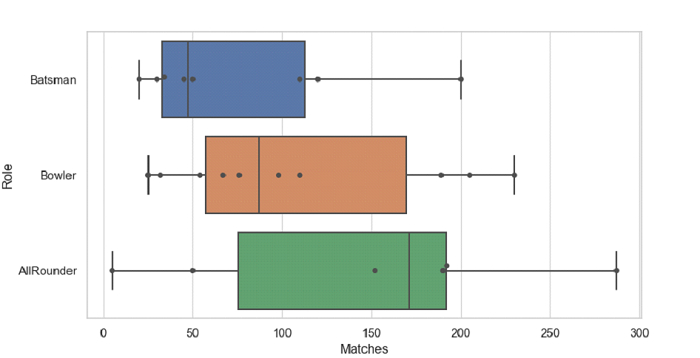# Python Pandas - Draw swarms of observations on top of a box plot with Seaborn

Swarm Plot in Seaborn is used to draw a categorical scatterplot with non-overlapping points. The seaborn.swarmplot() is used for this. Draw swarms of observations on top of a box plot using the seaborn.boxplot().

Let’s say the following is our dataset in the form of a CSV file −Cricketers2.csv

At first, import the required libraries −

import seaborn as sb
import pandas as pd
import numpy as np
import matplotlib.pyplot as plt

Load data from a CSV file into a Pandas DataFrame −

dataFrame = pd.read_csv("C:\Users\amit_\Desktop\Cricketers2.csv")


Draw swarms of observations on top of a box plot −

sb.boxplot(x = "Matches", y = "Role", data= dataFrame, whis=np.inf)
sb.swarmplot(x = "Matches", y = "Role", data= dataFrame, color=".3")

## Example

Following is the code

import seaborn as sb
import pandas as pd
import numpy as np
import matplotlib.pyplot as plt

# Load data from a CSV file into a Pandas DataFrame

sb.set_theme(style="whitegrid")

# draw swarms of observations on top of a box plot
sb.boxplot(x = "Matches", y = "Role", data= dataFrame, whis=np.inf)
sb.swarmplot(x = "Matches", y = "Role", data= dataFrame, color=".3")

# display
plt.show()

## Output

This will produce the following outputUpdated on: 27-Sep-2021

151 Views